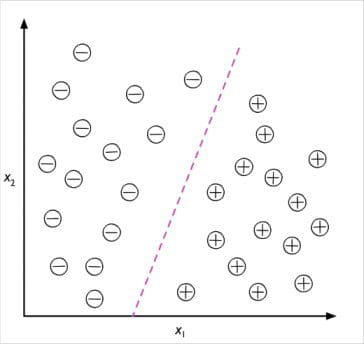Topics: AI | Data Science | Data Visualization | Deep Learning | Machine Learning | NLP | Python | R | Statistics

KDnuggets Home » News » 2017 » Nov » Tutorials, Overviews » 3 different types of machine learning ( 17:n43 )

# 3 different types of machine learning

In this extract from “Python Machine Learning” a top data scientist Sebastian Raschka explains 3 main types of machine learning: Supervised, Unsupervised and Reinforcement Learning. Use code PML250KDN to save 50% off the book cost.

If you’re new to machine learning it’s worth starting with the three core types: supervised learning, unsupervised learning, and reinforcement learning. In this tutorial, taken from the brand new edition of Python Machine Learning, we’ll take a closer look at what they are and the best types of problems each one can solve.

Learn more about the algorithms behind machine learning – and how to build them in Python Machine Learning 2nd Edition. This overview is an excerpt from this book.

use code PML250KDN to save 50% off the book cost.### Making predictions about the future with supervised learning

The main goal in supervised learning is to learn a model from labeled training data that allows us to make predictions about unseen or future data. Here, the term supervised refers to a set of samples where the desired output signals (labels) are already known.Considering the example of email spam filtering, we can train a model using a supervised machine learning algorithm on a corpus of labeled emails, emails that are correctly marked as spam or not-spam, to predict whether a new email belongs to either of the two categories. A supervised learning task with discrete class labels, such as in the previous email spam filtering example, is also called a classification task. Another subcategory of supervised learning is regression, where the outcome signal is a continuous value:

#### Classification for predicting class labels

Classification is a subcategory of supervised learning where the goal is to predict the categorical class labels of new instances, based on past observations. Those class labels are discrete, unordered values that can be understood as the group memberships of the instances. The previously mentioned example of email spam detection represents a typical example of a binary classification task, where the machine learning algorithm learns a set of rules in order to distinguish between two possible classes: spam and non-spam emails.

However, the set of class labels does not have to be of a binary nature. The predictive model learned by a supervised learning algorithm can assign any class label that was presented in the training dataset to a new, unlabeled instance. A typical example of a multiclass classification task is handwritten character recognition. Here, we could collect a training dataset that consists of multiple handwritten examples of each letter in the alphabet. Now, if a user provides a new handwritten character via an input device, our predictive model will be able to predict the correct letter in the alphabet with certain accuracy. However, our machine learning system would be unable to correctly recognize any of the digits zero to nine, for example, if they were not part of our training dataset.

The following figure illustrates the concept of a binary classification task given 30 training samples; 15 training samples are labeled as negative class (minus signs) and 15 training samples are labeled as positive class (plus signs). In this scenario, our dataset is two-dimensional, which means that each sample has two values associated with it: X1 and X2

Now, we can use a supervised machine learning algorithm to learn a rule—the decision boundary represented as a dashed line—that can separate those two classes and classify new data into each of those two categories given its X1 and X2 values:### Regression for predicting continuous outcomes

Classification allows us to assign categorical, unordered labels to instances. A second type of supervised learning is the prediction of continuous outcomes, which is also called regression analysis. In regression analysis, we are given a number of predictor (explanatory) variables and a continuous response variable (outcome or target), and we try to find a relationship between those variables that allows us to predict an outcome.

For example, let’s assume that we are interested in predicting the math SAT scores of our students. If there is a relationship between the time spent studying for the test and the final scores, we could use it as training data to learn a model that uses the study time to predict the test scores of future students who are planning to take this test.

The following figure illustrates the concept of linear regression. Given a predictor variable x and a response variable y, we fit a straight line to this data that minimizes the distance—most commonly the average squared distance—between the sample points and the fitted line. We can now use the intercept and slope learned from this data to predict the outcome variable of new data:Top Stories Past 30 Days
Most Popular
Most SharedGet KDnuggets, a leading newsletter on AI, Data Science, and Machine Learning